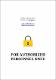Please use this identifier to cite or link to this item: `https://scidar.kg.ac.rs/handle/123456789/11106`
 Title: Degree-based energies of graphs Authors: das, kinkarGutman, IvanMilovanović I.Milovanovíc E.Furtula, BorisIssue Date: 2018 Abstract: © 2018 Elsevier Inc. Let G=(V,E) be a simple graph of order n and size m, with vertex set V(G)={v1,v2,…,vn}, without isolated vertices and sequence of vertex degrees Δ=d1≥d2≥⋯≥dn=δ>0, di=dG(vi). If the vertices vi and vj are adjacent, we denote it as vivj∈E(G) or i∼j. With TI we denote a topological index that can be represented as TI=TI(G)=∑i∼jF(di,dj), where F is an appropriately chosen function with the property F(x,y)=F(y,x). A general extended adjacency matrix A=(aij) of G is defined as aij=F(di,dj) if the vertices vi and vj are adjacent, and aij=0 otherwise. Denote by fi, i=1,2,…,n the eigenvalues of A. The “energy” of the general extended adjacency matrix is defined as ETI=ETI(G)=∑i=1n|fi|. Lower and upper bounds on ETI are obtained. By means of the present approach a plethora of earlier established results can be obtained as special cases. URI: https://scidar.kg.ac.rs/handle/123456789/11106 Type: article DOI: 10.1016/j.laa.2018.05.027 ISSN: 0024-3795 SCOPUS: 2-s2.0-85048493950 Appears in Collections: Faculty of Science, Kragujevac#### Page views(s)

119

29.86 kBAdobe PDF